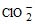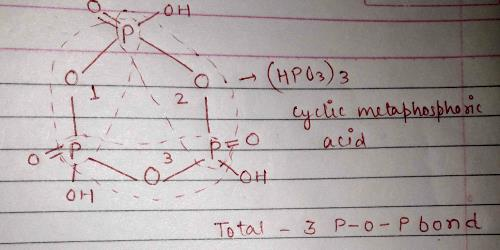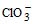Courses

# MCQ (Previous Year Questions) - P Block (Level 2)

## 25 Questions MCQ Test Chemistry for JEE Advanced | MCQ (Previous Year Questions) - P Block (Level 2)

Description
This mock test of MCQ (Previous Year Questions) - P Block (Level 2) for JEE helps you for every JEE entrance exam. This contains 25 Multiple Choice Questions for JEE MCQ (Previous Year Questions) - P Block (Level 2) (mcq) to study with solutions a complete question bank. The solved questions answers in this MCQ (Previous Year Questions) - P Block (Level 2) quiz give you a good mix of easy questions and tough questions. JEE students definitely take this MCQ (Previous Year Questions) - P Block (Level 2) exercise for a better result in the exam. You can find other MCQ (Previous Year Questions) - P Block (Level 2) extra questions, long questions & short questions for JEE on EduRev as well by searching above.
QUESTION: 1

Solution:
QUESTION: 2

### Nitrogen is liberated by the thermal decomposition of -       [IIT-1991]

Solution: Nitrogen is liberated by the thermal decomposition of ammonium nitrite (NH4NO2),NaN3,(NH4)2 Cr2O7
QUESTION: 3

### There is no S – S bond in -                [IIT-1991]

Solution:
QUESTION: 4

The type of hybrid orbitals used by chlorine atom inion.          [IIT-1992]

Solution:
We can calculate the number of orbitals involved in hybridisation by using the reaction.
Number of orbital involved in hybridisation by using the relation:
Number of orbital involved in hybridisation =1/2 (V + M − C + A)
Where V =  number of valence electrons
M =  number of monovalent atoms surrounding the atom
C = Charge on cation.
A = Charge on anion.
Number of orbitals involved in hybridisation = 1/2 (7 + 0 + 0 + 1) = 4
Since 4 orbitals are involved in hybridisation so it is SP^3 hybridised.
Hence A is the correct answer.
QUESTION: 5

STATEMENT-I

Although PF5, PCl5 and PBrare known, the pentahalides of nitrogen have not been observed

STATEMENT-II

Phosphorus has lower electronegativity than nitrogen.            [IIT-1994]

Solution:
QUESTION: 6

In P4O10 each P atom is linked with............O atoms -

Solution:
QUESTION: 7

Which one of the following species is not a pseudohalide ?

Solution:
QUESTION: 8

Which of the following statements is correct for CsBr3 ?         [IIT-1996]

Solution:
QUESTION: 9

Hydrolysis of one mole of peroxodisulphuric acid produces– [IIT-1996]

Solution: H2S2S8 + H2O → H2SO4 + H2SO5 ( peroxomonosulphuric acid)
QUESTION: 10

KF combines with HF to form KHF2. The compound contains the species :       [IIT-1996]

Solution:
KHF2 → K^+  + HF2^−
Hence, KHF2 contains K^+ and HF​2^-. HF2^- ion is formed by hydrogen bonding between F^- ion and HF molecule.
So, the correct answer is (c)
QUESTION: 11

White phosphorus (P4) has -      [IIT-1998]

Solution:
QUESTION: 12

In Compound of type ECl3, where E = B, P As or Bi, the angles Cl – E – Cl for different E are in the order              [IIT-1999]

Solution:
QUESTION: 13

On heating ammonium dichromate, the gas evolved is           [IIT-1999]

Solution: When you heat Ammonium Chromate, a green insoluble residue is seen which is Cr2O3. The further products which are seen are Steam and Nitrogen gas
QUESTION: 14

One mole of calcium phosphide on reaction with excess of water gives-     [IIT-1999]

Solution:
QUESTION: 15

The oxidation numbers of sulphur in S8, S2F2, H2S, respectively, are →       [IIT-1999]

Solution:
QUESTION: 16

Ammonia can be dried by -        [IIT-2000]

Solution:
QUESTION: 17

The number of P – O – P bonds in cyclic metaphosphoric acid is - [IIT-2000]

Solution:According to the above structure, there are three P-O-P bonds.

Hence A is the correct answer.

QUESTION: 18

Amongst H2O, H2S, H2Se and H2Te, the one with the highest boiling point is     [IIT-2000]

Solution:
QUESTION: 19

The number of S – S bonds in sulphur trioxide trimer (S3O9) is –       [IIT- 2001]

Solution:
QUESTION: 20

Read the following statement and explanation and answer as per the option given below :      [IIT-2001]

Statement-I : F atom has a less negative electron gain enthalpy affinity than Cl atom.

Statement-II : Additional electrons are repelled more effectively by 3 p electrons in Cl atom than by 2p electron in F atom

Solution:
QUESTION: 21

The set with correct order of acidity is       [IIT- 2001]

Solution:
QUESTION: 22

The reaction 3 CIO_(aq ) →(aq) + 2 Cl_ (aq) is an example of      [IIT-2001]

Solution:
QUESTION: 23

H3PO4 and H3PO3 is -     [IIT-2003]

Solution:
QUESTION: 24

The acid having O–O bond is-      [IIT-2004]

Solution:
QUESTION: 25

Which phosphorus is thermodynamically most stable ?        [IIT-2005]

Solution: